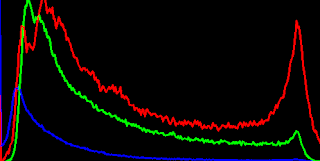## 12/28/2017

### calcHist for RGB image, opencv histogram example

A Example source code for rgb histogram, the source code uses calcHist function in opencv.

Important things in the source code are the part of split rgb mat to vector and drawing part.
Read the code carefully, so then you can understand easliy. ^^

inputthe result of rgb histogram<gist code start>

<gist code end>

reference : https://docs.opencv.org/2.4/doc/tutorials/imgproc/histograms/histogram_calculation/histogram_calculation.html